## RD Sharma Class 9 Solutions Chapter 20 Surface Areas and Volume of A Right Circular Cone Ex 20.2

These Solutions are part of RD Sharma Class 9 Solutions. Here we have given RD Sharma Class 9 Solutions Chapter 20 Surface Areas and Volume of A Right Circular Cone Ex 20.2

Other Exercises

Question 1.
Find the volume of a right circular cone with:
(i) radius 6 cm, height 7 cm
(ii) radius 3.5 cm, height 12 cm
(iii) height 21 cm and slant height 28 cm. [NCERT]
Solution:
(i) Radius of a cone (r) = 6 cm
and height (h) = 7 cm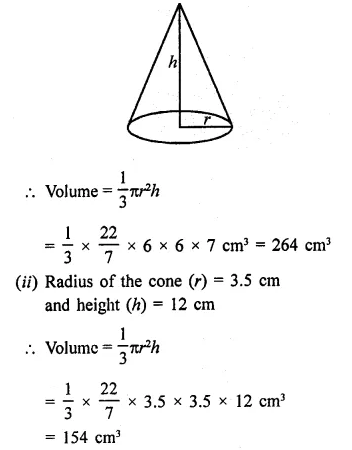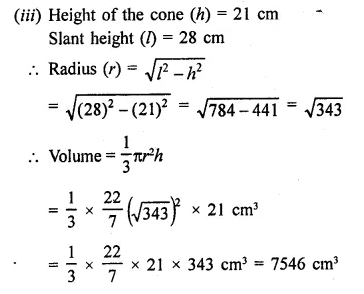Question 2.
Find the capacity in litres of a conical vessel with:
(i) radius 7 cm, slant height 25 cm,
(ii) height 12 cm, slant height 13 cm. [NCERT]
Solution:
(i) Radius of the conical vessel (r) = 7 cm
Slant height (h) = 25 cm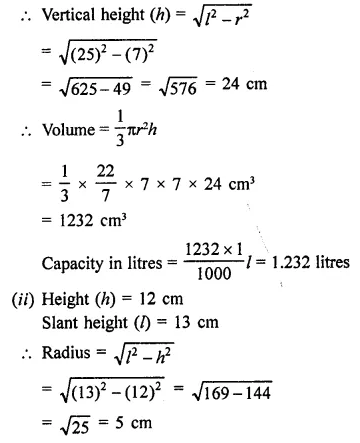Question 3.
Two cones have their heights in the ratio 1 : 3 and the radii of their bases in the ratio 3:1. Find the ratio of their volumes.
Solution:
Ratio in the heights of two cones =1:3
and ratio in their radii = 3: 1
Let radius of first cone (r1) = x
and of second cone (r2) = 3x
and height of first cone (h1) = 3y
and of second cone (h2)Question 4.
The radius and the height of a right circular cone are in the ratio 5 : 12. If its volume is 314 cubic metre, find the slant height and the radius (Use π = 3.14).
Solution:
Ratio in the radius and height of a cone = 5 : 12
Volume = 314 cm3
and height (h) = 12x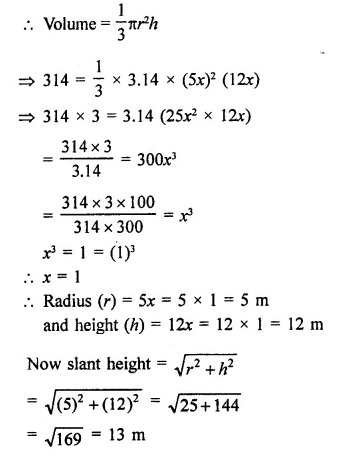Question 5.
The radius and height of a right circular cone are in the ratio 5 : 12 and its volume is 2512 cubic cm. Find the slant height and radius of the cone. (Use π = 3.14).
Solution:
Ratio in the radius and height of a right circular cone = 5 : 12
Volume = 2512 cm3
Then height (h) = 12x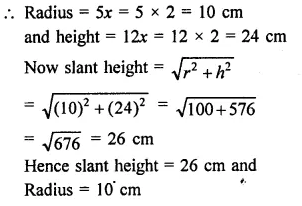Question 6.
The ratio of volumes of two cones is 4 : 5 and the ratio of the radii of their bases is 2:3. Find the ratio of their vertical heights.
Solution:
Ratio in volumes of two cones = 4:5
and ratio in radii = 2:3
Let radius of the first cone (r1) = 2x
Then radius of second cone (r2) = 3x
Let h1, h2 be their heights respectively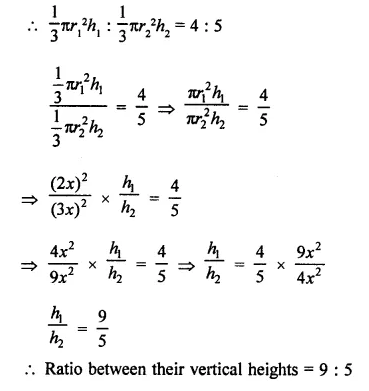Question 7.
Ratio between their vertical heights = 9:5 7. A cylinder and a cone have equal radii of their bases and equal heights. Show that their volumes are in the ratio 3:1.
Solution:
Let r be the radius and h be the height of a cylinder and a cone, then
Volume of cylinder = πr2hQuestion 8.
If the radius of the base of a cone is halved, keeping the height same, what is the ratio of the volume of the reduced cone to that of the original cone?
Solution:
Let r be the radius and h be the height of the cone, then
Volume = $$\frac { 1 }{ 3 }$$ πr2h
By halving the radius and same height theQuestion 9.
A heap of wheat is in the form of a cone of diameter 9 m and height 3.5 m. Find its volume. How much canvas cloth is required to just cover the heap? (Use π = 3.14). [NCERT]
Solution:
Diameter of conical heap of wheat = 9 m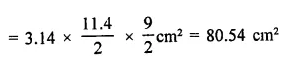Question 10.
Find the weight of a solid cone whose base is of diameter 14 cm and vertical height 51 cm, supposing the material of which it is made weighs 10 grams per cubic cm.
Solution:
Diameter of the base of solid cone = 14 cm
and vertical height (h) = 51 cm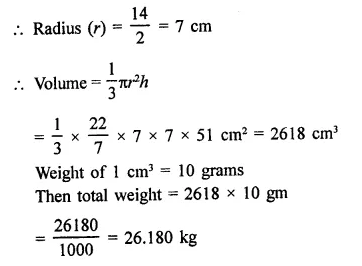Question 11.
A right angled triangle of which the sides containing the right angle are 6.3 cm and 10 cm in length, is made to turn round on the longer side. Find the volume of the solid, thus generated. Also, find its curved surface area.
Solution:
Length of sides of a right angled triangle are 6.3 cm and 10 cm
By turning around the longer side, a cone is formed in which radius (r) = 6.3 cm
and height (h) = 10 cm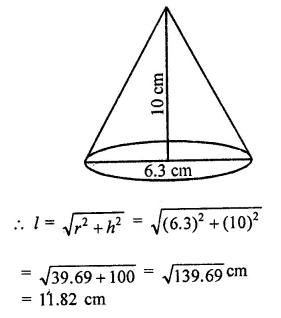Question 12.
Find the volume of the largest right circular cone that can be fitted in a cube whose edge is 14 cm.
Solution:
Side of cube = 14 cm ,
Radius of the largest cone that can be fitted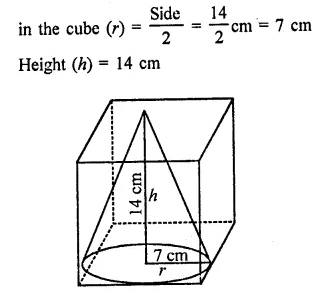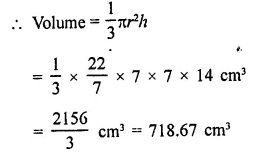Question 13.
The volume of a right circular cone is 9856 cm3. If the diameter of the base is 28 cm, find:
(i) height of the cone
(ii) slant height of the cone
(iii) curved surface area of the cone. [NCERT]
Solution:
Volume of a right circular cone = 9856 cm3
Diameter of the base = 28 cm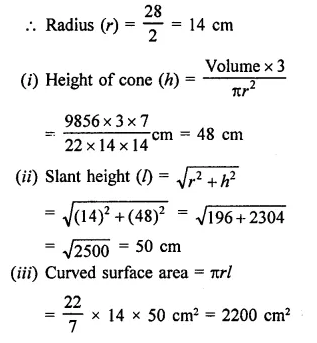Question 14.
A conical pit of top diameter 3.5 m is 12 m deep. What is its capacity in kilolitres? [NCERT]
Solution:
Diameter of the top of the conical pit = 3.5 m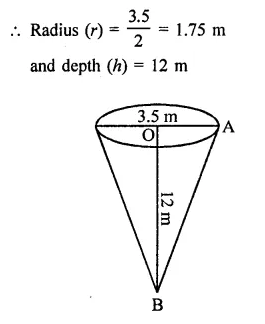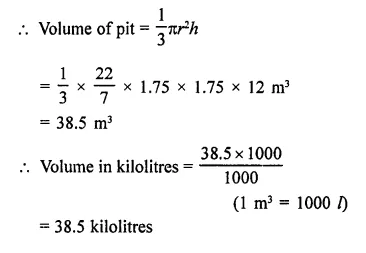Question 15.
Monica has a piece of Canvas whose area is 551 m2. She uses it to have a conical tent made, with a base radius of 7 m. Assuming that all the stitching margins and wastage incurred while cutting, amounts to approximately 1 m2. Find the volume of the tent that can be made with it. [NCERT]
Solution:
Area of Canvas = 551 m2
Area of wastage = 1 m2
Actual area = 551 – 1 = 550 m2
Base radius of the conical tent = 7 mLet l be the slant height and h be the vertical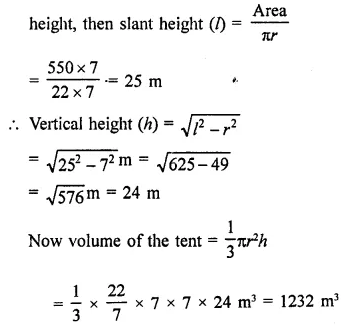Hope given RD Sharma Class 9 Solutions Chapter 20 Surface Areas and Volume of A Right Circular Cone Ex 20.2 are helpful to complete your math homework.

If you have any doubts, please comment below. Learn Insta try to provide online math tutoring for you.